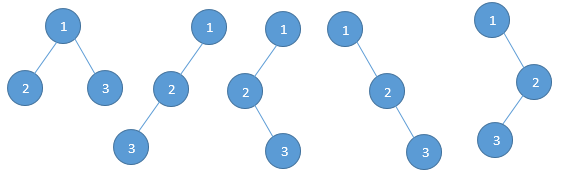Home Theory How many binary trees are possible with n nodes?

# How many binary trees are possible with n nodes?

Question: How many binary trees are possible with n nodes?
Input: Nodes = 3

For, example consider a tree with 3 nodes (n = 3), it will have a maximum combination of 5 different treesIn general, if there are n nodes, there exist (2n)!/(n+1)! different trees.

#### You may also likeJuly 26, 2017 - 17:44

Catalan Number is for BSTApril 28, 2017 - 01:22

no you are wrong the number of possible binary trees with 4 nodes is 14, but 2^4-4 is not 14.March 14, 2015 - 19:17

I think total number of binary trees possible for n nodes is equal to Catalan number
C(n) = (2n)! / ((n+1)! * n!)
for n = 4, 14 binary trees are possible as given by catalan number. While 2^n-n = 12. for n=4

http://en.wikipedia.org/wiki/Catalan_numberMarch 16, 2015 - 00:48

Hi Amit,
For a binary “search” tree, the possible combinations is C(n), the Catalan number, we are talking about binary trees in general.
Thanks for the extra information though. :)